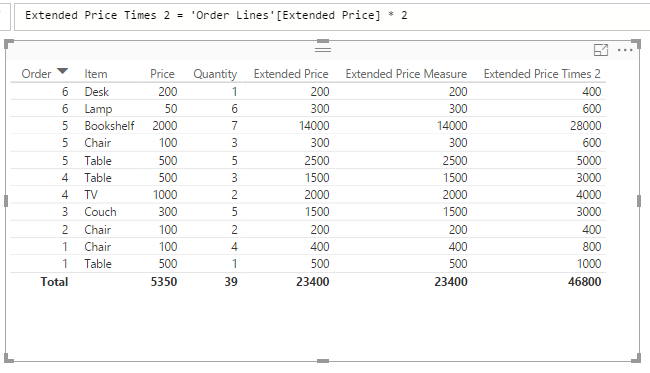# DAX Calculated Columns

Let’s start with a dataset that has sales orders. There are sales order lines: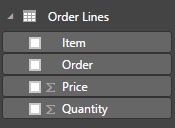And the data looks like this: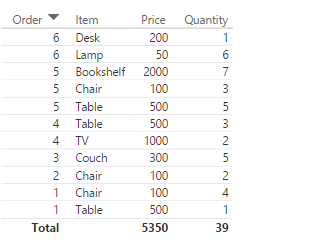However, there is no extended price in this dataset. To calculate it, let’s add a new calculated column. Click on the ellipse on the dataset: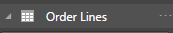And select New column: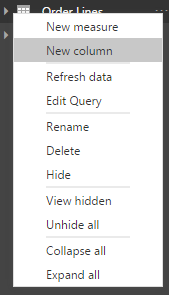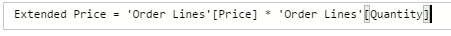The new column will appear in the dataset: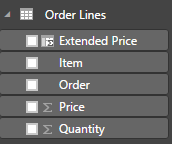And the new column can be added to our Power BI table: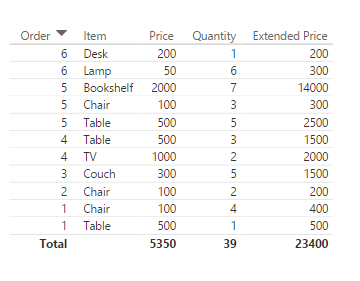You can then use that calculated column in measures: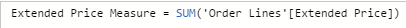And other calculated column calculations: